Equilibrium (2018 NHT) 1) Consider the following reaction.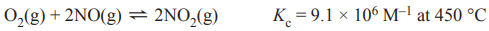Which one of the following statements is correct? A. Kc is very large; the reaction is reversible. B. Changing the pressure will affect the value of Kc . C. Kc is very large; the reaction is effectively irreversible. D. Changing the temperature will not affect the value of Kc . Solution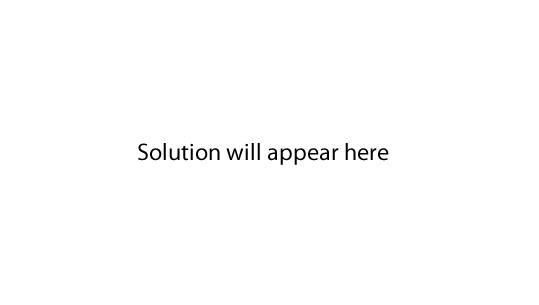2) The following reaction, in which dinitrogen tetroxide, N2O4, is converted to nitrogen dioxide, NO2, forms an equilibrium.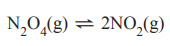At a given temperature, the equilibrium constant for this reaction is 3.15 M and the molar concentration of N2O4 at equilibrium is 0.350 M. At this temperature, the molar concentration of NO2 at equilibrium is A. 0.550 M B. 1.05 M C. 1.10 M D. 3.00 M Solution3) Dissolved carbon dioxide, CO2, can react with water, H2O, to form carbonic acid, H2CO3. H2CO3 can also react with H2O to form bicarbonate ions, HCO3–, and hydronium ions, H3O+ accordin gto the equations below.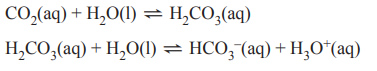A beaker that contains 2 L of deionised water is placed in a room and left overnight so that these two reactions reach equilibrium. In the morning one change is made to the system. Which one of the following changes is most likely to result in an increase in the concentration of H3O+ ions? A. Dilute the solution. B. Bubble in more CO2 gas. C. Add a few drops of phenolphthalein indicator. D. Add a few drops of 0.1 M sodium hydroxide, NaOH, solution. Solution4) Nitrosyl chloride, NOCl, is a highly toxic gas used in the chemical industry as an oxidising agent. The formation reaction of NOCl from nitrogen monoxide, NO, and chlorine, Cl2, is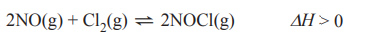This reaction forms an equilibrium above 100 °C. A scientist conducted two experiments on the equilibrium reaction of NOCl. The initial experiments were conducted in evacuated and sealed 4 L containers at 150 °C. Experiment 1 - 2 mol of NOCl was injected into a previously evacuated, sealed 4 L container. Experiment 2 - 4 mol of NOCl was injected into another previously evacuated, sealed 4 L container. a) Which experiment had the highest initial rate of production of Cl2 or were both rates equal? Justify your answer. Solutionb) If, for Experiment 1, the concentrations of NOCl and NO were equal at equilibrium, [NOCl] = [NO], then what conclusion could be made about the relative concentrations of NOCl and NO in Experiment 2 at equilibrium? Justify your answer. Solutionc) 2 mol of an inert gas is injected into the container in Experiment 1. The temperature is kept at 150 °C. What effect will this have on the rate of production of the Cl2 in the container? Circle the correct response below. Justify your answer using collision theory. i. decreases ii. no change iii. increases Solutiond) The temperature for Experiment 2 is increased to 200 °C. Explain the effect on the equilibrium concentration of NOCl in the reaction. Solution